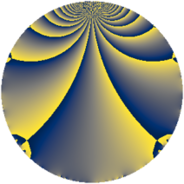# Properties

 Label 1045.2.aLevel $1045$ Weight $2$ Character orbit 1045.a Rep. character $\chi_{1045}(1,\cdot)$ Character field $\Q$ Dimension $59$ Newform subspaces $11$ Sturm bound $240$ Trace bound $5$

# Related objects

## Defining parameters

 Level: $$N$$ $$=$$ $$1045 = 5 \cdot 11 \cdot 19$$ Weight: $$k$$ $$=$$ $$2$$ Character orbit: $$[\chi]$$ $$=$$ 1045.a (trivial) Character field: $$\Q$$ Newform subspaces: $$11$$ Sturm bound: $$240$$ Trace bound: $$5$$ Distinguishing $$T_p$$: $$2$$

## Dimensions

The following table gives the dimensions of various subspaces of $$M_{2}(\Gamma_0(1045))$$.

Total New Old
Modular forms 124 59 65
Cusp forms 117 59 58
Eisenstein series 7 0 7

The following table gives the dimensions of the cuspidal new subspaces with specified eigenvalues for the Atkin-Lehner operators and the Fricke involution.

$$5$$$$11$$$$19$$FrickeDim.
$$+$$$$+$$$$+$$$$+$$$$7$$
$$+$$$$+$$$$-$$$$-$$$$9$$
$$+$$$$-$$$$+$$$$-$$$$7$$
$$+$$$$-$$$$-$$$$+$$$$5$$
$$-$$$$+$$$$+$$$$-$$$$8$$
$$-$$$$+$$$$-$$$$+$$$$6$$
$$-$$$$-$$$$+$$$$+$$$$8$$
$$-$$$$-$$$$-$$$$-$$$$9$$
Plus space$$+$$$$26$$
Minus space$$-$$$$33$$

## Trace form

 $$59 q - 3 q^{2} - 4 q^{3} + 53 q^{4} + 3 q^{5} + 20 q^{6} - 8 q^{7} + 9 q^{8} + 55 q^{9} + O(q^{10})$$ $$59 q - 3 q^{2} - 4 q^{3} + 53 q^{4} + 3 q^{5} + 20 q^{6} - 8 q^{7} + 9 q^{8} + 55 q^{9} - 3 q^{10} - q^{11} + 36 q^{12} - 14 q^{13} + 32 q^{14} - 4 q^{15} + 37 q^{16} - 18 q^{17} + 25 q^{18} - q^{19} + 13 q^{20} - 3 q^{22} + 52 q^{24} + 59 q^{25} - 10 q^{26} + 8 q^{27} - 56 q^{28} + 2 q^{29} - 4 q^{30} - 24 q^{31} + 25 q^{32} - 4 q^{33} - 46 q^{34} + 17 q^{36} - 38 q^{37} + 9 q^{38} + 16 q^{39} - 15 q^{40} - 18 q^{41} - 16 q^{42} - 4 q^{43} + q^{44} + 23 q^{45} - 16 q^{46} + 32 q^{47} + 28 q^{48} + 43 q^{49} - 3 q^{50} + 40 q^{51} - 26 q^{52} - 38 q^{53} + 48 q^{54} + 7 q^{55} + 8 q^{56} - 4 q^{57} + 22 q^{58} + 44 q^{59} - 28 q^{60} - 22 q^{61} - 32 q^{62} + 45 q^{64} - 6 q^{65} - 12 q^{66} + 4 q^{67} - 62 q^{68} - 48 q^{69} + 8 q^{70} - 32 q^{71} + 5 q^{72} - 58 q^{73} - 50 q^{74} - 4 q^{75} - 7 q^{76} + 16 q^{77} - 80 q^{78} - 56 q^{79} + 13 q^{80} + 67 q^{81} - 14 q^{82} + 68 q^{83} - 104 q^{84} - 10 q^{85} - 52 q^{86} - 16 q^{87} - 15 q^{88} - 18 q^{89} - 39 q^{90} - 32 q^{91} - 24 q^{92} + 32 q^{93} + 72 q^{94} - q^{95} + 4 q^{96} - 42 q^{97} - 67 q^{98} - 13 q^{99} + O(q^{100})$$

## Decomposition of $$S_{2}^{\mathrm{new}}(\Gamma_0(1045))$$ into newform subspaces

Label Dim $A$ Field CM Traces A-L signs $q$-expansion
$a_{2}$ $a_{3}$ $a_{5}$ $a_{7}$ 5 11 19
1045.2.a.a $1$ $8.344$ $$\Q$$ None $$-1$$ $$-2$$ $$-1$$ $$-2$$ $+$ $+$ $+$ $$q-q^{2}-2q^{3}-q^{4}-q^{5}+2q^{6}-2q^{7}+\cdots$$
1045.2.a.b $1$ $8.344$ $$\Q$$ None $$1$$ $$0$$ $$1$$ $$0$$ $-$ $+$ $-$ $$q+q^{2}-q^{4}+q^{5}-3q^{8}-3q^{9}+q^{10}+\cdots$$
1045.2.a.c $2$ $8.344$ $$\Q(\sqrt{2})$$ None $$2$$ $$4$$ $$2$$ $$0$$ $-$ $+$ $+$ $$q+(1+\beta )q^{2}+2q^{3}+(1+2\beta )q^{4}+q^{5}+\cdots$$
1045.2.a.d $5$ $8.344$ $$\Q(\zeta_{22})^+$$ None $$-3$$ $$-7$$ $$5$$ $$-11$$ $-$ $+$ $-$ $$q+(-1+\beta _{1}-\beta _{4})q^{2}+(-2-\beta _{2}+\beta _{3}+\cdots)q^{3}+\cdots$$
1045.2.a.e $5$ $8.344$ 5.5.36497.1 None $$-1$$ $$-3$$ $$-5$$ $$3$$ $+$ $-$ $-$ $$q+\beta _{4}q^{2}+(-1+\beta _{1})q^{3}+\beta _{3}q^{4}-q^{5}+\cdots$$
1045.2.a.f $6$ $8.344$ 6.6.7281497.1 None $$-2$$ $$-1$$ $$-6$$ $$5$$ $+$ $+$ $+$ $$q-\beta _{4}q^{2}+\beta _{3}q^{3}+(\beta _{4}+\beta _{5})q^{4}-q^{5}+\cdots$$
1045.2.a.g $6$ $8.344$ 6.6.131947641.1 None $$0$$ $$3$$ $$6$$ $$5$$ $-$ $+$ $+$ $$q+\beta _{1}q^{2}+(1-\beta _{3})q^{3}+(2+\beta _{2}-\beta _{3}+\cdots)q^{4}+\cdots$$
1045.2.a.h $7$ $8.344$ $$\mathbb{Q}[x]/(x^{7} - \cdots)$$ None $$1$$ $$3$$ $$-7$$ $$-1$$ $+$ $-$ $+$ $$q+\beta _{1}q^{2}+\beta _{5}q^{3}+(1+\beta _{2})q^{4}-q^{5}+\cdots$$
1045.2.a.i $8$ $8.344$ $$\mathbb{Q}[x]/(x^{8} - \cdots)$$ None $$-6$$ $$-7$$ $$8$$ $$-11$$ $-$ $-$ $+$ $$q+(-1+\beta _{1})q^{2}+(-1-\beta _{6})q^{3}+(2+\cdots)q^{4}+\cdots$$
1045.2.a.j $9$ $8.344$ $$\mathbb{Q}[x]/(x^{9} - \cdots)$$ None $$3$$ $$3$$ $$-9$$ $$-9$$ $+$ $+$ $-$ $$q-\beta _{1}q^{2}+\beta _{5}q^{3}+(1-\beta _{1}-\beta _{8})q^{4}+\cdots$$
1045.2.a.k $9$ $8.344$ $$\mathbb{Q}[x]/(x^{9} - \cdots)$$ None $$3$$ $$3$$ $$9$$ $$13$$ $-$ $-$ $-$ $$q+\beta _{1}q^{2}+\beta _{5}q^{3}+(1+\beta _{2})q^{4}+q^{5}+\cdots$$

## Decomposition of $$S_{2}^{\mathrm{old}}(\Gamma_0(1045))$$ into lower level spaces

$$S_{2}^{\mathrm{old}}(\Gamma_0(1045)) \cong$$ $$S_{2}^{\mathrm{new}}(\Gamma_0(11))$$$$^{\oplus 4}$$$$\oplus$$$$S_{2}^{\mathrm{new}}(\Gamma_0(19))$$$$^{\oplus 4}$$$$\oplus$$$$S_{2}^{\mathrm{new}}(\Gamma_0(55))$$$$^{\oplus 2}$$$$\oplus$$$$S_{2}^{\mathrm{new}}(\Gamma_0(95))$$$$^{\oplus 2}$$$$\oplus$$$$S_{2}^{\mathrm{new}}(\Gamma_0(209))$$$$^{\oplus 2}$$